# Solution November 18, 2007

### Problem

Let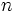$n\,$ be a natural number, such that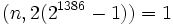$(n,2(2^{1386}-1))=1\,$. Let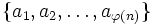$\{a_{1},a_{2},\dots,a_{\varphi(n)}\}$be a reduced residue system for$n\,$. Prove that: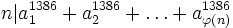$n|a_{1}^{1386}+a_{2}^{1386}+\dots+a_{\varphi(n)}^{1386}$

### Solution

Let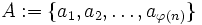$A := \{a_{1},a_{2},\dots,a_{\varphi(n)}\}$. Modulo$n\,$, we have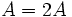$A = 2A\,$, because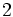$2\,$ is coprime to$n\,$. Therefore,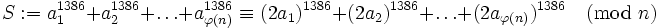$S := a_{1}^{1386}+a_{2}^{1386}+\dots+a_{\varphi(n)}^{1386} \equiv (2a_{1})^{1386}+(2a_{2})^{1386}+\dots+(2a_{\varphi(n)})^{1386} \pmod n$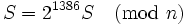$S = 2^{1386} S \pmod n\,$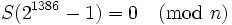$S (2^{1386}-1) = 0 \pmod n\,$

But$n\,$ is also coprime to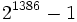$2^{1386}-1\,$ so we can divide that equivalence by$2^{1386}-1\,$. This gives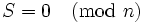$S = 0 \pmod n\,$

which is what we wanted to prove.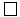$\square$